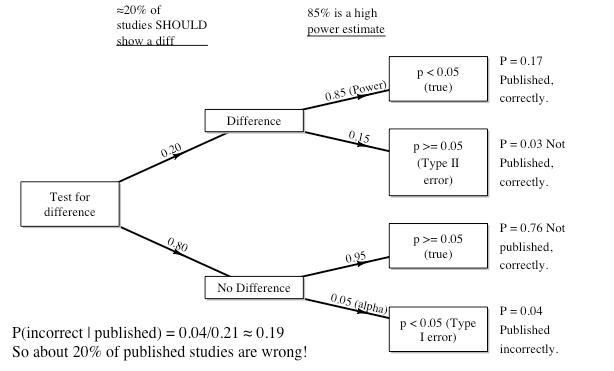# How likely is any given study to be “true”? A conditional probability / inference exploration.

An important point I try to get across to students is that statistics is real math, but by its very nature statistical techniques always have a possibility of error. Which means that sometimes an experiment will find a “statistically significant” result (and therefore, in a super-simplistic view, result in a publishable paper in a respected journal) simply through random variation. And also, there are major issues with how academic publishing uses and abuses statistical techniques.

To explore this idea for myself I made this diagram:This is a gross oversimplification, of course, and it requires two completely made-up assumptions – the 20% and the 85% are both estimates I pulled out of nowhere. But honestly, it wouldn’t surprise me if both of them are a bit high, meaning that 19% of published studies being wrong number is probably too LOW. I think there’s a lesson or two for intro / high school / AP Statistics just using this tree diagram. I could give a simplified version that doesn’t have the probabilities or error names and ask students to identify the correct location for alpha, Type I Error, Type II errror, and (average) power. We could have a discussion about what values seem reasonable for the two assumption points (the 20% and 85%) and calculate the % of erroneous studies. We could add a replication step – if the study is repeated at each branch (as they theoretically should be in a perfect world), how does the 20% change? It also leads to an interesting discussion about things that seem totally reasonable but actually can break things even more – for example, if published studies are NOT repeated but unpublished ones ARE (because the original scientists still believe there should be a change and so try again) what happens then?

This could be a really good segue into this article: 100 Psychology experiments repeated, less than half successful.